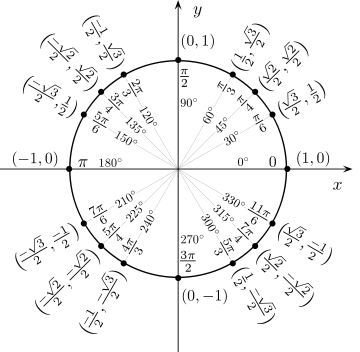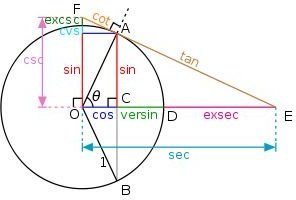# Trigonometry Cheat Sheet: Ace your Exam with This Study Guide

Before you start reading, you should know that this is only a review. If you don’t have any experience with the subject, there is not enough information here to teach it to you. For those of you that have studied the material before, this is a condensed formula sheet to help you prepare for exams. It is also helpful as a handy homework reference.

## Angles

Until now, you probably measured angles in degrees. Most of trig uses radians.

Unit Circle

The unit circle is segmented into 360 degrees or 2*pi radians. (For this article, p will denote pi=3.14159…)

90 degrees = p/2 radians

180 degrees = p radians

0 degrees = 0 radians = 2p radians = 360 degrees (Note that 0 and 2p are often used interchangeably)

The unit circle below gives the commonly used angles and their sines/cosines.If Θ is an angle made by connecting (0,0), (1,0) and any other point on the unit circle M = (a,b), then cosΘ = a, sinΘ = b, or equivalently: (a,b) = (cosΘ, sinΘ)

For example: the line for Θ=p/4 radians crosses the circle at (sqrt(2)/2, sqrt(2)/2). Therefore x = cos Θ = sqrt(2)/2 = y =sinΘ, or equivalently: (sqrt(2)/2, sqrt(2)/2) = (cos(p/4), sin(p/4)).

Negative Angles

Counterclockwise movement on the unit circle is measured in positive units and clockwise movement is measured in negative units. Therefore, the distance from p to 2p can also be notated as 0 to -p, depending on which way you travel the circle.

Relationships between angles are important:

Supplementary Angles: x+y = p = 90 degrees

Complementary Angles: x+y = 2p = 180 degrees

## Triangles

For the purpose of the trig cheat sheet, and conventionally, if the sides of a triangle are labeled a, b, c then the angles are ABC, where A is the angle made by b and c, B is the angle made by a and c, and C is the angle made by a and b. A is called the opposite angle of a, and a is the opposite side of A.

Much of trigonometry is based on triangles, usually right or acute.

Triangle area formulas:

½*base*height=Area

½*a*b*sinC=Area

½*b*c*sinA=Area

½*c*a*sinB=Area

s*(s-a)*(s-b)*(s-c)=(a+b+c)*(b+c)*(a+c)*(a+b)=Area^2 [s=a+b+c]

## More With Triangles

This trig cheat sheet uses Θ, A and B to represent angles. Your notes or textbook may use x and y or other variables, but the meanings are the same.

Right Triangle and Sine, Cosine, Tangent

An easy pneumonic for the sine, cosine, tangent right triangle definitions is SOHCAHTOA. This stands for Sine=Opposite/Hypotenuse (SOH); Cosine=Adjacent/Hypotenuse (COH); Tangent=Opposite/Adjacent (TOA).

The chart below gives a breakdown of the trig functions and their relationship to right triangles and the unit circle.Here is a simple way to remember the right triangle relationships: Hold up your left hand, palm facing out, fingers together and thumb sticking out the right like an L. Now, imagine a line from the tip of your forefinger to the tip of your thumb. If you pretend the curve where your thumb meets your hand is a right angle, then you have a right triangle. Your thumb is a, your forefinger is b, and the imaginary line (the hypotenuse) is c. Then the angle at the tip of your forefinger is A, the tip of your thumb is B and the right angle is C. Now, use that to memorize the following definitions:

sinA=opp/hyp = a/c

cosA=adj/hyp= b/c

tanA=opp/adj = a/b

cscA = hyp/opp = c/a = 1/sinA

secA = hyp/adj = c/b = 1/cosA

cotA = adj/opp = c/b = 1/tanA

## Laws and Theorems

The trig cheat sheet only gives the formulas for these theorems. If you are required to use the full definition, or are unsure when to use each formula, you will need additional study material.

Pythagorean Theorem, Law of Sines, Law of Cosines, Law of Tangents are all about the relationships between the sides and angles of triangles.

~Pythagorean theorem (only for right triangles with sides a, b, c, where c is the hypotenuse):

Using the definition of a right triangle on your hand (as explained above) the lengths of the sides are related by the following formula:

a2 +b2 = c2

~Law of sines (for all triangles with sides lengths a, b, c and with angles A, B, C)

sin(A)/a = sin(B)/b = sin(C)/c

~Law of cosines (for all triangles with sides lengths a, b, c and with angles A, B, C)

c²=a²+b²-2*a*b*cos(C)

b²=a²+c²-2*a*c*cos(B)

a²=b²+c²-2*b*c*cos(A)

When the angle C is p, 0, or 2p, the law of cosines reduces to a simpler formula. If it is easier for you to memorize these too, go ahead, but usually I just solve for them.

When C = p: c²=a²+b² (the Pythagorean theorem)

When C=0, cos(0)=1: c2 = (a-b)2

When C=2p, cos(2)=-1: c 2 = (a+b)2

~Law of Tangents (for all triangles with sides a, b, c and with angles A, B, C)

(a+b)/(a-b) = [tan(1/2(A+B))]/[tan(1/2(A-B))]

## Important Trig Formulas (More Memorization)

Here is a list of the most important trig formulas. It is best to memorize as many as you can, but remember that some can be derived from others (if that’s easier for you) and many are related. The trig cheat sheet uses Greek letters such as Θ to represent angles. Please note that where marked below, a and b are angles, and p represents pi=3.14159….

Squared formulas

sin2(Θ)+cos2(Θ)=1

1+tan2(Θ) = sec2(Θ)

1+cot2(Θ) = csc2(Θ)

Symmetry

sin(-Θ) = -sinΘ

cos(-Θ) = cosΘ

tan(-Θ) = -tanΘ

Periodic Functions (p = pi = 3.14159…)

sin(Θ) = sin(Θ+2kp)

cos(Θ) = cos(Θ+2kp)

where k is any integer, positive or negative.

Sum and Difference formulas (a and b are angles)

sin(a+b)=sin(a)cos(b)+sin(b)cos(a)

cos(a+b)=cos(a)cos(b)-sin(a)sin(b)

tan(a+b)=[tan(a)+tan(b)]/[1-tan(a)tan(b)]

sin(a-b)=sin(a)cos(b)-sin(b)cos(a)

cos(a-b)=cos(a)cos(b)+sin(a)sin(b)

tan(a-b)=[tan(a)-tan(b)]/[1+tan(a)tan(b)]

tan([a+b]/2) = [sin(a)+sin(b)]/[cos(a)+cos(b)] = -[cos(a)-cos(b)]/[sin(a)-sin(b)]

Power Reduction Formulas

sin2(Θ) = [1-cos(2Θ)]/2

cos2(Θ) = [1+cos(2Θ)]/2

Product to Sum Formulas (a and b are angles)

cos(a)cos(b) = ½[cos(a-b) + cos(a+b)]

sin(a)sinb() = ½ [cos(a-b) – cos(a+b)]

sin(a)cos(b) = ½[sin(a+b) + sin(a-b)]

cos(a)sin(b) = ½[sin(a+b) – sin(a-b)]

Sum to Product Formulas (a and b are angles)

sin(a)+sin(b) = 2sin([a+b]/2)cos([a+b]/2)

cos(a)+cos(b) = 2cos([a+b]/2)cos([a-b]/2)

cos(a)-cos(b) = -2sin([a+b]/2)sin([a-b]/2)

Half, Double, and Triple Angle formulas (SQRT(arg) means take the square root of arg)

~Half Angle

sin(Θ/2) = SQRT([1-cos(Θ)]/2)

cos(Θ/2) = SQRT([1+cos(Θ)]/2)

tan(Θ/2) = sin(Θ)/[1+cos(Θ)] = [1-cos(Θ)]/sin(Θ)

~Double Angle

sin(2Θ)=2sin(Θ)cos(Θ) = 2tan(Θ)/[1+tan2(Θ)]

cos(2Θ)=cos2(Θ)-sin2(Θ) = 2cos2(Θ) – 1 = 1-2sin2(Θ) = [1-tan2(Θ)]/[1+tan2(Θ)]

tan(2Θ)=2tan(Θ)/[1-tan2(Θ)]

~Triple Angle

sin(3Θ) = 3sin(Θ)-4sin3(Θ)

cos(3Θ) = 4cos3(Θ) – 3cos(Θ)

tan(3Θ) = [3tan(Θ) – tan3(Θ)]/[1-3tan2(Θ)]

## This post is part of the series: Trig Help

Everything you need to know to get through your trig class. Whether in high school or college, the tips, tricks, formulas and methods you need can all be found here.